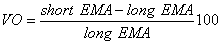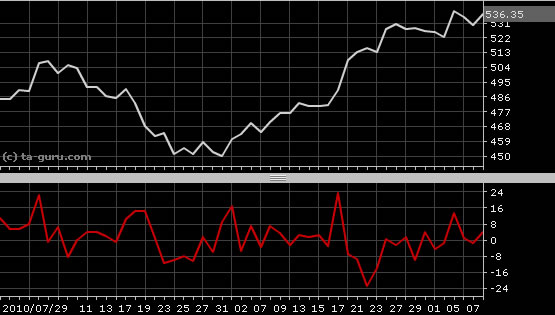Chart Pro online
User name (email):

 Recently used

 Forex

 Indices

# Volume Oscillator (VO)

Volume Oscillator (VO) is technical analysis indicator used for identifying periods of expanding or contracting volume.

Volume oscillator is calculated by formula:Volume oscillator is based on exponential moving average. For short EMA period is usually used value of 9 and for long EMA value of 26.Volume Oscillator (VO)

Interpretation

Volume oscillator oscillates around zero. Positive values indicate that volume levels are greater than average and relatively high.

Example